# 回文树

## 结构¶## 建造¶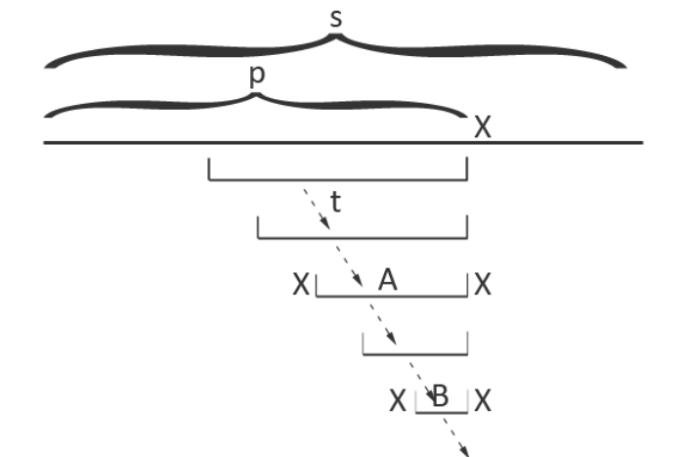## 线性状态数证明¶

• 时， 只有一个字符，同时也只有一个子串，并且这个子串是回文的，因此结论成立。

• 时，设 ，其中 表示 最后增加一个字符 后形成的字符串，假设结论对 串成立。考虑以最后一个字符 结尾的回文子串，假设它们的左端点由小到大排序为 。由于 是回文串，因此对于所有位置 ，有 。所以，对于 已经在 中出现过。因此，每次增加一个字符，本质不同的回文子串个数最多增加 个。

## 应用¶

### 回文子串出现次数¶

#include <bits/stdc++.h>
using namespace std;
typedef long long ll;
const int maxn = 300000 + 5;
namespace pam {
int sz, tot, last;
int cnt[maxn], ch[maxn], len[maxn], fail[maxn];
char s[maxn];
int node(int l) {
sz++;
memset(ch[sz], 0, sizeof(ch[sz]));
len[sz] = l;
fail[sz] = cnt[sz] = 0;
return sz;
}
void clear() {
sz = -1;
last = 0;
s[tot = 0] = '$'; node(0); node(-1); fail = 1; } int getfail(int x) { while (s[tot - len[x] - 1] != s[tot]) x = fail[x]; return x; } void insert(char c) { s[++tot] = c; int now = getfail(last); if (!ch[now][c - 'a']) { int x = node(len[now] + 2); fail[x] = ch[getfail(fail[now])][c - 'a']; ch[now][c - 'a'] = x; } last = ch[now][c - 'a']; cnt[last]++; } ll solve() { ll ans = 0; for (int i = sz; i >= 0; i--) { cnt[fail[i]] += cnt[i]; } for (int i = 1; i <= sz; i++) { ans = max(ans, 1ll * len[i] * cnt[i]); } return ans; } } // namespace pam char s[maxn]; int main() { pam::clear(); scanf("%s", s + 1); for (int i = 1; s[i]; i++) { pam::insert(s[i]); } printf("%lld\n", pam::solve()); return 0; }  ### 最小回文划分¶ 给定一个字符串 ，求最小的 ，使得存在 ，满足 均为回文串，且 依次连接后得到的字符串等于 考虑动态规划，记 表示 长度为 的前缀的最小划分数，转移只需要枚举以第 个字符结尾的所有回文串 由于一个字符串最多会有 个回文子串，因此上述算法的时间复杂度为 ，无法接受，为了优化转移过程，下面给出一些引理。 记字符串 长度为 的前缀为 ，长度为 的后缀为 周期：若 ，就称 的周期。 border：若 ，就称 的 border。 周期和 border 的关系： 的 border，当且仅当 的周期。 证明： 的 border，那么 ，因此 ，所以 就是 的周期。 周期，则 ，因此 ，所以 的 border。 引理 是回文串 的后缀， 的 border 当且仅当 是回文串。 证明： 对于 ，由 为回文串，因此有 ，所以 的 border。 对于 ，由 的 border，有 ，由 是回文串，有 ，因此 ，所以 是回文串。 下图中，相同颜色的位置表示字符对应相同。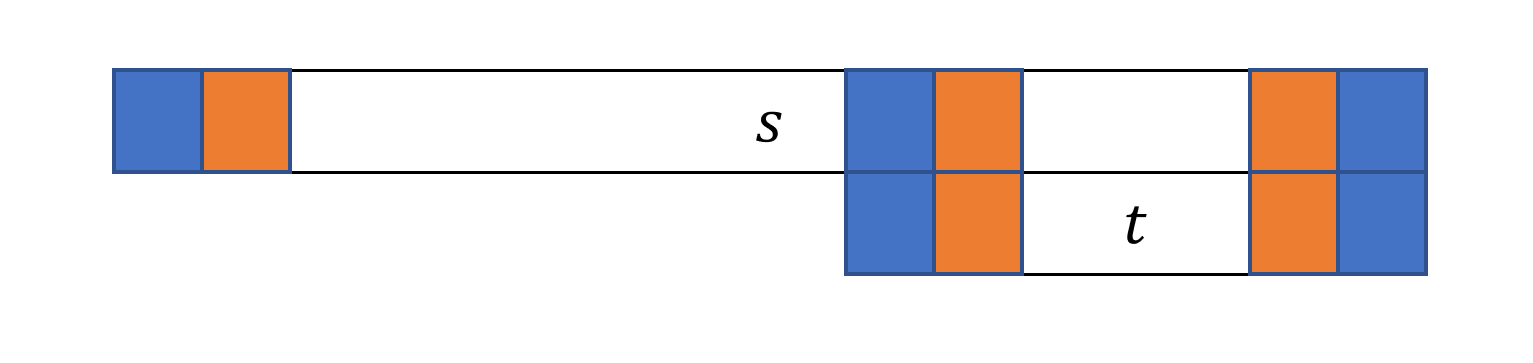引理 是回文串 的 border ( )， 是回文串当且仅当 是回文串。 证明： 是回文串，由引理 也是回文串。 是回文串，由 的 border，因此 ，因为 ，所以 也是回文串。 引理 是回文串 的 border，则 的周期， 的最小周期，当且仅当 的最长回文真后缀。 引理 是一个回文串， 的最长回文真后缀， 的最长回文真后缀。令 分别为满足 的字符串，则有下面三条性质 1. 如果 ，那么 2. 如果 ，那么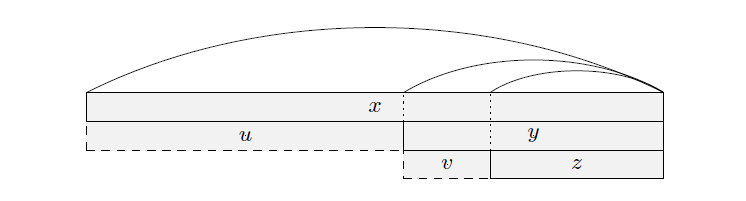证明： 1. 由引理 的推论， 的最小周期， 的最小周期。考虑反证法，假设 ，因为 的后缀，所以 既是 的周期，也是 的周期，而 的最小周期，矛盾。所以 2. 因为 的 border，所以 的前缀，设字符串 ，满足 （如下图所示），其中 的 border。考虑反证法，假设 ，那么 ，所以由引理 是回文串，由引理 的 border，又因为 ，所以 ，矛盾。所以 3. 都是 的前缀， ，所以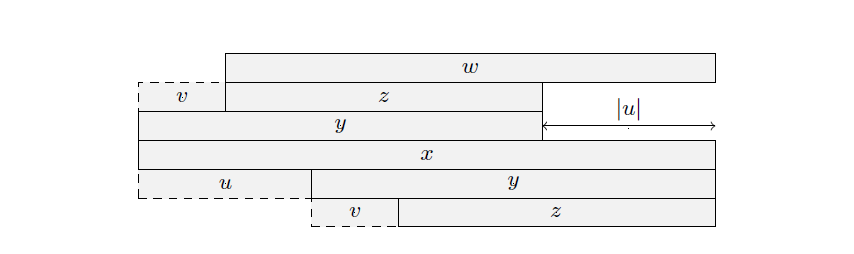推论： 的所有回文后缀按照长度排序后，可以划分成 段等差数列。 证明： 的所有回文后缀长度从小到大排序为 。对于任意 ，若 ，则 构成一个等差数列。否则 ，由引理 ，有 ，且 。因此，若相邻两对回文后缀的长度之差发生变化，那么这个最大长度一定会相对于最小长度翻一倍。显然，长度翻倍最多只会发生 次，也就是 的回文后缀长度可以划分成 段等差数列。 该推论也可以通过使用弱周期引理，对 的最长回文后缀的所有 border 按照长度 分类， ，考虑这 组内每组的最长 border 进行证明。详细证明可以参考金策的《字符串算法选讲》和陈孙立的 2019 年 IOI 国家候选队论文《子串周期查询问题的相关算法及其应用》。 有了这个结论后，我们现在可以考虑如何优化 的转移。 回文树上的每个节点 需要多维护两个信息， 表示节点 所代表的回文串的长度差，即 表示 一直沿着 fail 向上跳到第一个节点 ，使得 ，也就是 所在等差数列中长度最小的那个节点。 根据上面证明的结论，如果使用 指针向上跳的话，每向后填加一个字符，只需要向上跳 次。因此，可以考虑将一个等差数列表示的所有回文串的 值之和（在原问题中指 ），记录到最长的那一个回文串对应节点上。 表示 所在等差数列的 值之和，且 是这个等差数列中长度最长的节点，则 ，这里 是当前枚举到的下标。 下面我们考虑如何更新 数组和 数组。以下图为例，假设当前枚举到第 个字符，回文树上对应节点为 为橙色三个位置的 值之和（最短的回文串 算在下一个等差数列中）。 上一次出现位置是 （在 处结束）， 包含的 值是蓝色位置。因此， 实际上等于 和多出来一个位置的 值之和，多出来的位置是 。最后再用 去更新 ，这部分等差数列的贡献就计算完毕了，不断跳 ，重复这个过程即可。具体实现方式可参考例题代码。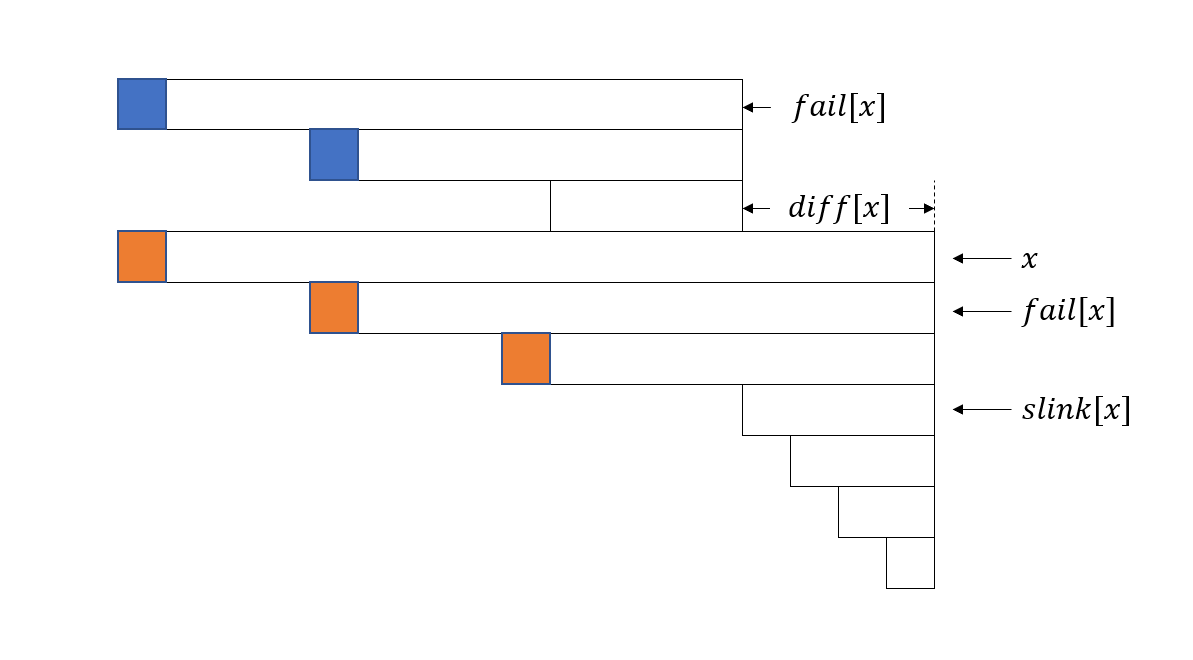最后，上述做法的正确性依赖于：如果 属于同一个等差数列，那么 上一次出现位置是 证明： 根据引理 的 border，因此其在 处出现。 假设 中的 位置出现。由于 属于同一个等差数列，因此 。多余的 处的 有交集，记交集为 ，设串 满足 。用类似引理 的方式可以证明， 是回文串，而 的前缀 也是回文串，这与 的最长回文前缀（后缀）矛盾。 给定一个字符串 ，要求将 划分为 ，其中 是偶数，且 ，求这样的划分方案数。 题解 构造字符串 ，问题等价于求 的偶回文划分方案数，把上面的转移方程改成求和形式并且只在偶数位置更新 数组即可。时间复杂度 ，空间复杂度 参考代码 #include <bits/stdc++.h> using namespace std; typedef long long ll; const int mod = 1e9 + 7; const int maxn = 1000000 + 5; inline int add(int x, int y) { x += y; return x >= mod ? x -= mod : x; } namespace pam { int sz, tot, last; int ch[maxn], len[maxn], fail[maxn]; int cnt[maxn], dep[maxn], dif[maxn], slink[maxn]; char s[maxn]; int node(int l) { sz++; memset(ch[sz], 0, sizeof(ch[sz])); len[sz] = l; fail[sz] = 0; cnt[sz] = 0; dep[sz] = 0; return sz; } void clear() { sz = -1; last = 0; s[tot = 0] = '$';
node(0);
node(-1);
fail = 1;
}
int getfail(int x) {
while (s[tot - len[x] - 1] != s[tot]) x = fail[x];
return x;
}
void insert(char c) {
s[++tot] = c;
int now = getfail(last);
if (!ch[now][c - 'a']) {
int x = node(len[now] + 2);
fail[x] = ch[getfail(fail[now])][c - 'a'];
dep[x] = dep[fail[x]] + 1;
ch[now][c - 'a'] = x;
dif[x] = len[x] - len[fail[x]];
if (dif[x] == dif[fail[x]])
else
}
last = ch[now][c - 'a'];
cnt[last]++;
}
}  // namespace pam
using pam::dif;
using pam::fail;
using pam::len;
int n, dp[maxn], g[maxn];
char s[maxn], t[maxn];
int main() {
pam::clear();
scanf("%s", s + 1);
n = strlen(s + 1);
for (int i = 1, j = 0; i <= n; i++) t[++j] = s[i], t[++j] = s[n - i + 1];
dp = 1;
for (int i = 1; i <= n; i++) {
pam::insert(t[i]);
for (int x = pam::last; x > 1; x = slink[x]) {
g[x] = dp[i - len[slink[x]] - dif[x]];
if (dif[x] == dif[fail[x]]) g[x] = add(g[x], g[fail[x]]);
if (i % 2 == 0) dp[i] = add(dp[i], g[x]);
}
}
printf("%d", dp[n]);
return 0;
}


## 相关资料¶

• 2017 年 IOI 国家候选队论文集 回文树及其应用 翁文涛

• 2019 年 IOI 国家候选队论文集 子串周期查询问题的相关算法及其应用 陈孙立

• 字符串算法选讲 金策